# Op amp 741 IC or Operational Amplifier 741 IC

The operational amplifier or op amp is an electronic device or element, that behaves like a voltage controlled voltage source. An op amp is a complex electronic device, which consists of resistors, capacitors, transistors and diodes. It is commercially available in integrated circuit form.

Most commonly available and used op amp IC is IC 741.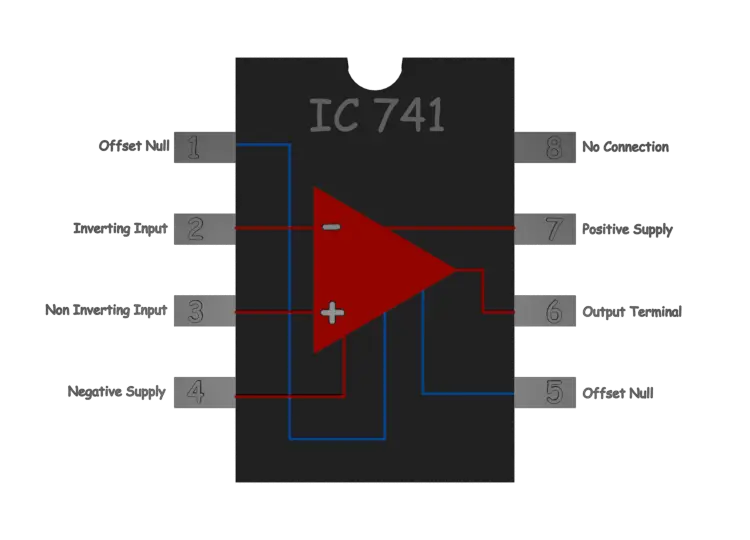The IC 741 is a small chip. It comprises eight pins. 2, 3 and 6 numbered pins are most significant.
Pin 2 is inverting terminal.
Pin 3 is non-inverting terminal.
Pin 6 is output terminal.
IC 741 mainly performs mathematical operations like addition, subtraction, division, multiplication, integration, differentiation etc. IC 741 has three stages such as differential input, gain, and push-pull output.
Pin 1 and 5 are “offset null” or “balance” terminals.
The op amp is nothing but a differential amplifier. When we apply the same voltage at the inverting terminal (Pin 2) and the non-inverting terminal (Pin 3) there should not be any voltage at the output terminal (Pin 6). This condition is an ideal. Practically there is always a small voltage (offset voltage) appears at pin 6 even the voltage applied at pin 2 and 3 are exactly equal. Offset voltage appears at output because we can not make the parameters of transistors and value of biasing resistances of differential amplifier perfectly same.

But still, we can make the output voltage exactly zero if we apply the offset voltage to the inputs to balance the output offset voltage. We call this as the input offset voltage.
We can add some external circuitries to reduce the mismatches in the IC 741. These circuitries balance the offset voltage. IC 741 has two terminals (Pin 1 and Pin 5) at input stage for the purpose where we can add external circuits to balance offset voltages. Offset null adjustment usually requires a potentiometer with its slider connected to the negative supply.

Pin 4 is for negative power supply and pin 7 is for positive power supply.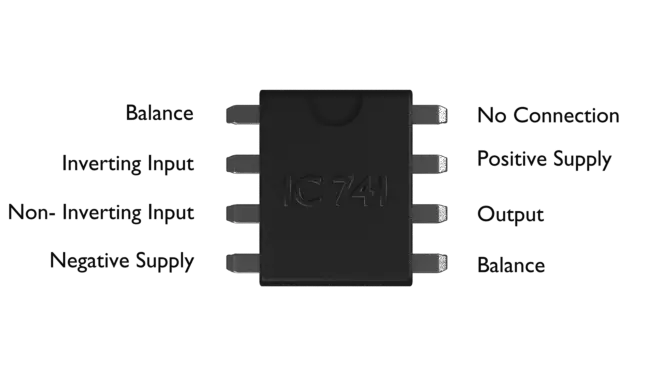1. Pin 1 is balance pin (offset null)
2. Pin 2 is inverting input
3. Pin 3 is non inverting input
4. Pin 4 is negative power supply pin
5. Pin 5 is balance pin (offset null)
6. Pin 6 is output pin
7. Pin 7 is positive power supply pin
8. Pin 8 does not have any connection

We furnish the typical parameters of a 741 IC in the table below.

 Parameters Typical Range Ideal Value Open Loop Gain(A) 105 to 108 ∝ Input Resistance(Ri) 105 to 1013 ∝ Output Resistance(Ro) 10 to 100 0

A typical range of supply voltage in 741 IC is,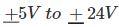The output of an op amp can not cross this range of supply voltage. That means, output voltage v0 ranges from – Vcc to + Vcc. If we denote the supply voltage as Vcc then, mathematically we can write,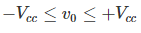That means, output voltage v0 of an op amp cannot reach below –Vcc and above Vcc. Inside this range v0 is linearly proportional to the input differential voltage vd. This can be graphically represented as,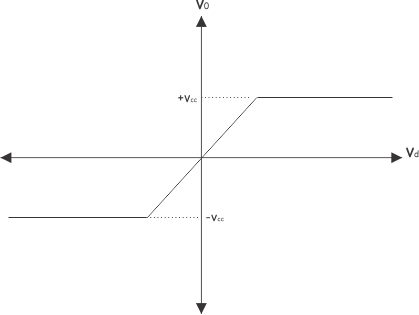An op amp is intended to be operated in that linear region.

Want To Learn Faster? 🎓
Get electrical articles delivered to your inbox every week.
No credit card required—it’s 100% free.